## Finding an interval for rational numbers with a high denominator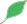This article is a stub.This means that it cannot be considered to contain or lead to any mathematically interesting information.

### Quick description

Some problems require one to find an interval of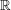in which rational numbers, when written in lowest terms as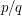, have a denominator that is greater than a certain number. This sort of problem can be difficult to approach because given an interval, it may be hard to visualise what rational numbers lie in it. However, given the right notation the solution to this sort of problem becomes almost intuitive.

### Prerequisites

Definition of continuity, basic facts about real and rational numbers, intervals of real numbers, neighbourhoods.

### Example 1

Every rational numbercan be written in the form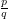, where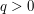and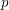andare integers without any common divisors. Consider the function defined onby: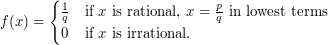(This is Thomae's function). Prove that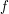is continuous at all irrational numbers and discontinuous at all rational numbers.